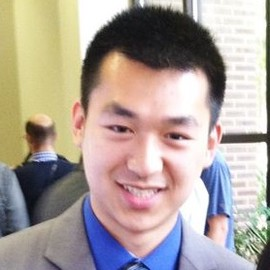# Automated Curriculum Learning for Neural Networks

Graves et al., 2017

## Summary

• Maximize learning efficiency by following a curriculum
• Measure of the amount that the network learns from each data sample used as reward
• Nonstationary multi-armed bandit algorithm
• Consider a variety of signals based on rate of increase in prediction accuracy and network complexity
• Experimental results with LSTMs on three curricula
• Links: [ website ] [ pdf ]

## Background

• Based on the idea that a curriculum of tasks of increasing difficulty can accelerate learning
• Curriculum learning is highly sensitive to the exact progression through tasks (e.g. when to switch to next task, return to old tasks, what is the measure of difficulty etc.)

## Methods

• Treat picking next task as stochastic policy, optimized for maximizing learning progress

• Progress signal is evaluated for each training example

• Nonstationary multi-armed bandit

• task is a distribution D over batches from $X := (A \times B)^N$, where $A$ and $B$ are the set of inputs and outputs, respectively

• curriculum is an esemble of task $D_1, …, D_N$

• syllabus is a time-varying sequence of distributions over tasks

• View curriculum containing N tasks as an N-armed bandit (Exp3 algorithm Auer et al. 2002)

• Adaptively rescale rewards to [-1,1] based on highest and lowest quantiles using reservoir sampling

• Learning progress signals

• Policy should maximize rate at which model minimizes the loss, should be reflected in reward
• Loss-driven progress

• Prediction gain (PG) - change in loss for a sample, before and after training on it
• Gradient prediction gain (GPG) - first-order approximation to prediction gain. Avoids addition forward pass of PG
• Self prediction gain (SPG) - change in loss for a new sample from the same task
• Target prediction gain (TPG) - change in loss for a new sample from the target task
• Mean prediction gain (MPG) - change in loss for a new sample from all tasks
• Complexity progress signals

• Based on Minimum Description Length principle - tradeoff between model complexity and data compression
• Variational complexity gain (VCG) - change in model complexity induced by sample
• Gradient variational complexity gain (GVCG) - first order approximation to VCG
• L2 gain (L2G) - change in l2 norm of model parameters
• Bias-variance tradeoff for different progress signals.

## Results

• Synthetic N-gram language modelling

• character-level n-gram model on Bible data
• n from 0 to 10
• For each model, generate dataset of 1M characters, divided into 150 characters disjoint sequences.
• LSTM trained to predict last 100 characters from first 50
• Intuitively, higher n produces more structure and should have higher learning progress
• Complexity progress signals quickly result in a strong focus on the 10-gram task
• Except VCG
• Loss based signals trend towards higher n, but are slower
• Less so in PG, GPG
• Repeat Copy

• Network is trained to repeat a random sequence a given number of times
• Difficulty based on length of sequence and number of repeats, both vary from 1 to 13 for this experiment (169 tasks in total)
• ML training
• PG, SPG, TPG slightly faster than uniform
• L2G, GL2G, GPG slower
• VI training
• GVCG faster than uniform
• VCG slower
• However, training on target task failed to learn, indicating need for curriculum
• One example showed GVCG syllabus first focus on short sequences with high repeats, then long sequences with low repeats.
• Loss is reduced in many tasks that the policy does not focus on
• bAbI

• Created larger dataset of 1M sotires for each of the 20 tasks
• PG, SPG better than uniform, others worse
• VI training worse than uniform

## Conclusion

• Best learning progress signal depends on the task
• Some may perform worse than uniform policy
• PG for ML training and GVCG for VI training generally most consistent
• Both only rely on current sample
• Curriculum composed of a small number of very similar tasks